## Introduction

Let us understand the need for a new collection of numbers - rational numbers.

Did you know, even decimal numbers are rational numbers? Can you think of a day-to-day activity where you use decimals? Check today’s temperature on your phone – is it in decimals? Most probably yes! Temperatures are written as rational numbers. For example, the temperature in India is currently around 36.7°C. In Antarctica, the temperature is −10°C. Both temperatures are rational numbers. The image below shows the temperature of a region in Fahrenheit.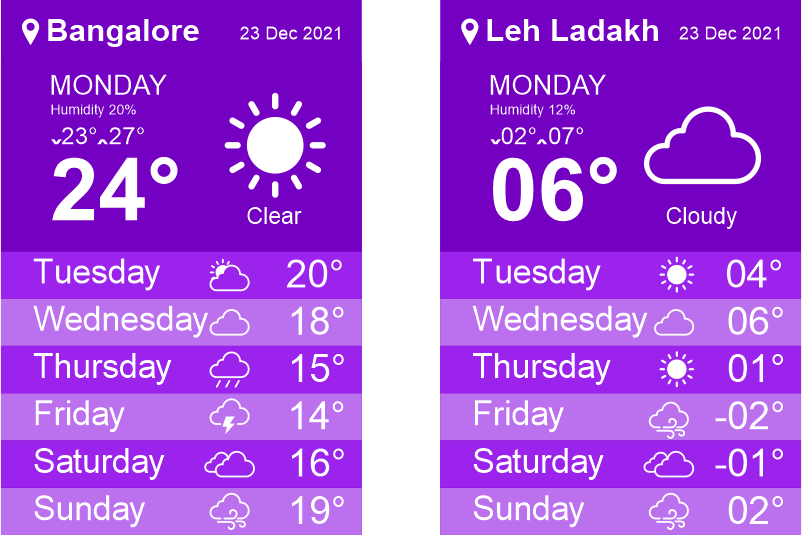All the temperatures indicated in this weather report are rational numbers.

#### Concepts

The chapter ‘Rational Numbers’ covers the following concepts:

#### What Are Rational Numbers

Can we say that -1 3 is a fraction? Here, -1 3 is of the form a b , but the numerator is an integer. Hence, it will not belong to the group of fractions. What do we call such numbers as? We need a different number system to represent such numbers.

We call this system of numbers as rational numbers.

A rational number is a number that can be written in the form p q , where ‘p’ and ‘q’ are integers and q≠0. For example, a b is a rational number since (-1) and 3 are integers and 3 ≠ 0.

Look at the representation below for better understanding.The word ‘rational’ arises from the term ‘ratio’. We know that a ratio 3 : 2 can also be written as 3 2 . Here, 3 and 2 are natural numbers. Similarly, the ratio of two integers p and q (q≠0), i.e., p : q can be written in the form p q .

This is the form in which rational numbers are expressed.

We know what a numerator and a denominator of a fraction is. Let us learn about the denominator and numerator of rational numbers.

In the rational number p q , 'p' is called as numerator and 'q' is called as denominator.

Rational numbers include integers and fractions.

From the previous example, we see that if we multiply both the numerator and denominator by the same number then the value of the number remains the same.

Here, -14 8 and 21 -12 are equivalent rational numbers of the rational number -7 4 .

Rational numbers with different numerators and denominators that represent the same value are called as equivalent rational numbers.

We can get the equivalent rational numbers by multiplying the numerator and denominator of a rational number by the same non - zero integer. Can we also get equivalent rational numbers by dividing the same non- zero integer? Consider the rational number -8 20 . Let us divide the numerator and denominator by 4.

-8÷4 20÷4 = -2 5

Also, we can divide the numerator and denominator by 2.

-8÷2 20÷2 = -4 10

Here, we can see that -8 20 = -2 5 = -4 10

These are the equivalent rational numbers of -8 20 .

Hence, we can find equivalent rational numbers by dividing the numerator and denominator by the same non- zero integer.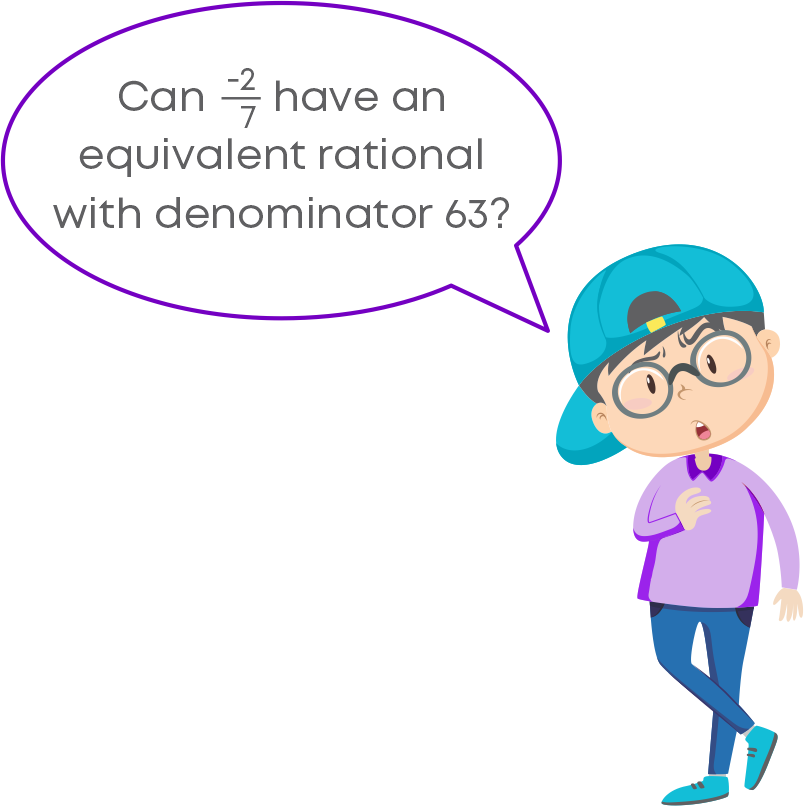We can write this as: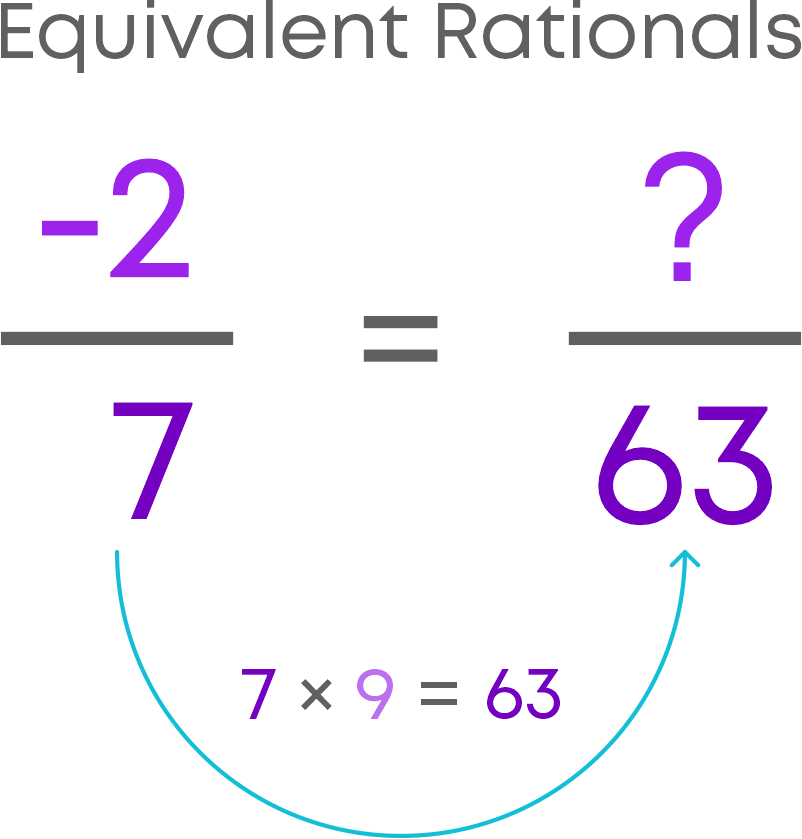Since the denominator 7 was multiplied by 9 to get the equivalent rational number, the numerator (-2) also should be multiplied by the same number, i.e., 9.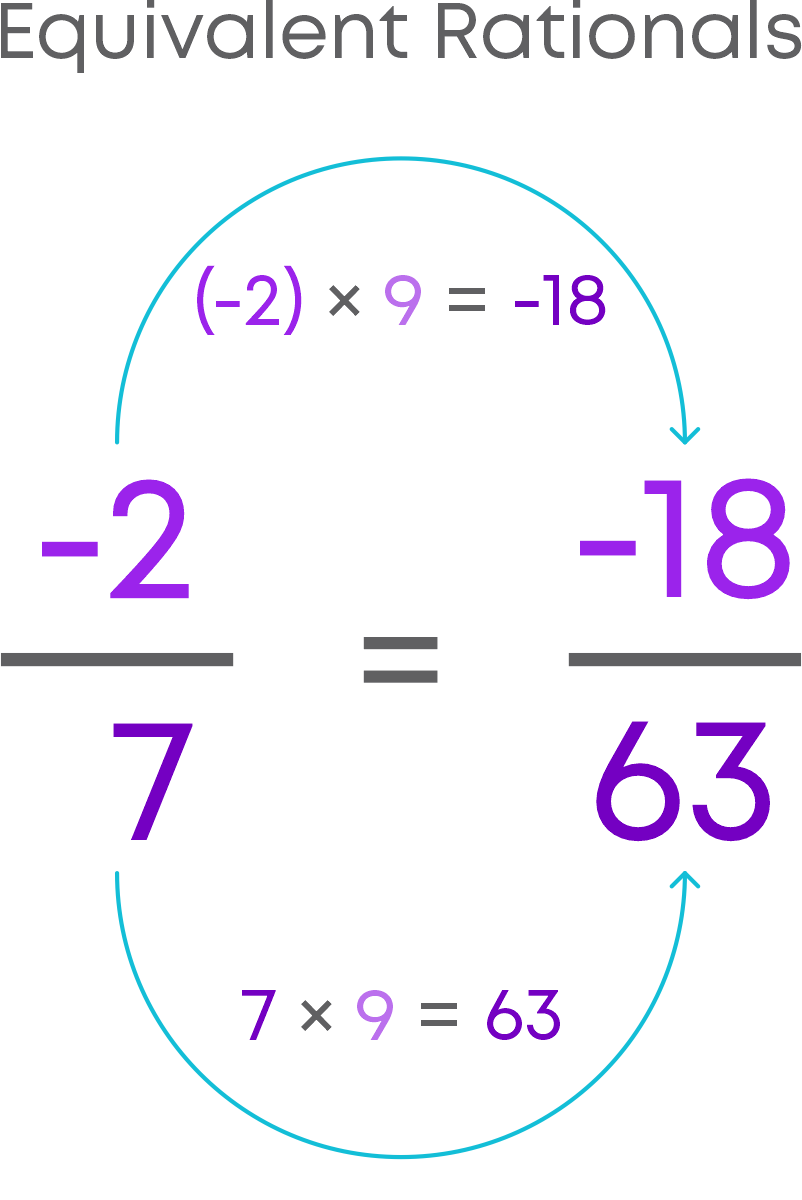Hence, the equivalent rational number of -2 7 with denominator 63 is -18 63 .

Consider the rational number 4 7 , here the numerator 4 and denominator 7 both are positive integers. These are called as positive rational numbers.

Now, consider the rational number -4 -7 . Here, both numerator and denominator are negative integers.

Consider the rational number -4 7 , the numerator (-4) is a negative integer and the denominator 7 is positive integer. These are called as negative rational numbers.

Now, consider the rational number 4 -7 , here, numerator is a positive integer and denominator is a negative integer.

Remember! Positive rational numbers – numerator and denominator have the same signs. Negative rational number – numerator and denominator have different signs.

We know how to identify whole numbers and fractions based on their position on the number line. On a horizontal number line, positive integers lie to the right of 0 and negative integers to the left of 0. In the same manner, on a horizontal number line,

• Positive rational numbers lie to the right of 0.
• Negative rational numbers lie to the left of 0.

Let us now identify rational numbers on the number line. Identifying positive rational numbers on a number line is the same as identifying fractions on a number line. Consider the number line:We can see that the length between 0 and 1 is divided into two equal parts. 0 is nothing but 0 2 . From 0, let us move towards right. The first division is 1 2 . Now, let us consider the number line given below.Here we can see that the length between ‘0’ and 1 is divided into four equal parts. 0 is nothing but 0 4 . The first division is 1 4 , the second division is 2 4 , the third division is 3 4 .1 is the fourth division which represents 4 5 . From this, we can see that the denominator of the rational number indicates the number of parts that each unit should be divided into.

Positive rational numbers lie to the right of 0 and similarly, negative rational numbers lie to the left of 0 on a horizontal number line.

A rational number is said to be in the standard form or simplest if its denominator is a positive integer and the numerator and denominator have no common factor other than 1. For example, 3 5 , -7 9 , 5 8 , -7 5 .

We can convert a given fraction to simplest form by dividing the numerator and denominator by their H.C.F. In a similar way, we can convert a given rational number to its simplest form. Let us convert the rational number -12 15 to its simplest form.

Step 1: Find the HCF of the numerator and the denominator by ignoring the negative sign.

12 = 2 × 2 × 3

15 = 3 × 5

HCF of 12 and 15 is 3.

Step 2: Divide the numerator and the denominator of the rational number by their HCF.

-12÷3 15÷3 = -4 5

Hence, the simplest form of -12 15 is -4 5 .

#### Comparison Of Rational Numbers

Let us consider the two positive rational numbers 1 2 and 2 3 . To represent the given rational numbers on the number line, first let us convert them as rational numbers having the same denominator. For this, we first must find the LCM of the denominators.

LCM of 2 and 3 is 6.

1×3 2×3 = 3 6 and 2×2 3×2 = 4 6

Now, we can represent them on the number line.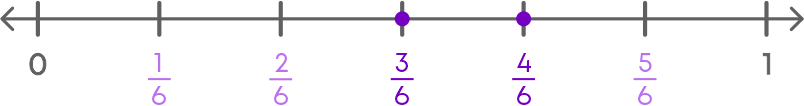Here, we can see that the rational number 3 6 lies to the left of 4 6 .

So, 3 6 is less than 4 6 . Hence, 1 2 is lesser than 2 3 .

Rational numbers can be compared without using a number line as well. For example, consider the rational numbers used above, 1 2 and 2 3 . After converting them to fractions with the same denominator, i.e., 3 6 and 4 6 , we can see that 3 < 4. Hence, 1 2 is lesser than 2 3 .

Let us understand how to compare two negative rational numbers. Consider the rational numbers -1 4 and -1 8 . We can plot these on the number line, by converting each rational number to have the same denominator.

For this, we find the LCM of the denominators and then find the equivalent of each rational number with LCM as the denominator.

LCM of 4 and 8 is 8.

-1×2 4×2 = -2 8 and -1×1 8×1 = -1 8

Now, we can represent them on the number line.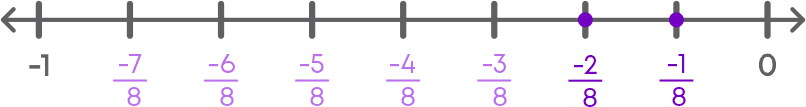The rational number to the left of the number line is always less than the rational number to the right.

Clearly, -2 8 < -1 8

Hence, -1 4 < -1 8 .

You can compare negative rational numbers without using a number line by comparing the numerators of the fractions after converting them to fractions with the same denominator.

Remember, to compare two negative rational numbers, we can compare them ignoring their negative signs and then reverse the order.

We know that negative integers are always less than the positive integers. Also, a negative rational number lies to the left of zero. In the same manner, a negative rational number is less than a positive rational number.

We know how to compare two rational numbers. Ordering rational numbers means arranging them in ascending or descending order. We can order the rational numbers in the same way as we have done for unlike fractions.

To order the rational numbers, 2 5 , 7 3 , -11 6 , and -7 12 , follow the steps below:

LCM of 5, 3, 6 and 12 = 60

2×12 5×12 = 24 60

7×20 3×20 = 140 60

-11×10 6×10 = -110 60

-7×5 12×5 = -35 60

We can see that -110 60 < -35 60 < 24 60 < 140 60

The rational numbers arranged in ascending order are as follows:

-11 6 , -7 12 , 2 5 , 7 3

The rational numbers arranged in descending order are as follows:

7 3 , 2 5 , -7 12 , -11 6

#### Rational Numbers Between Two Rational Numbers

Is there a definite number of rational numbers between two rational numbers? Let us find out.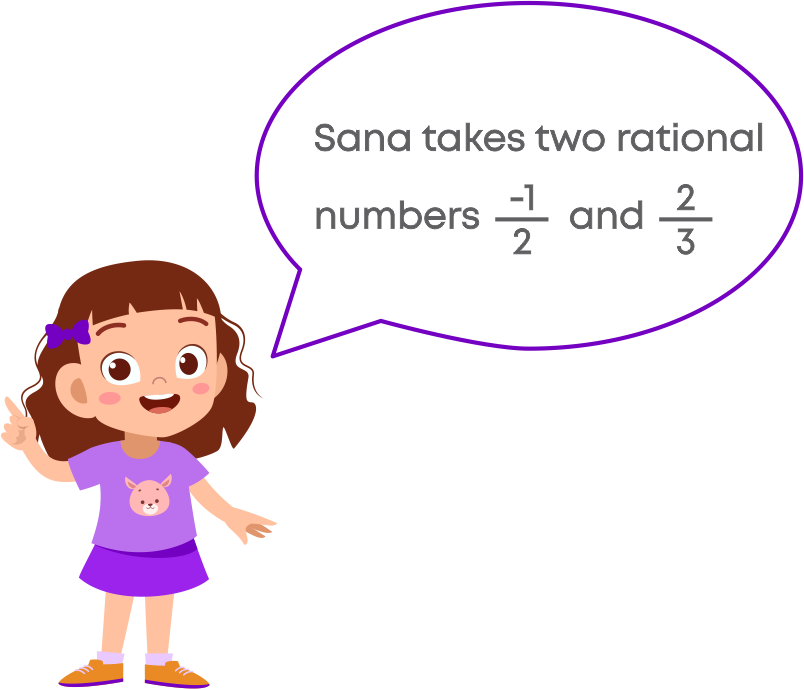First, she converts them as equivalent rational numbers by taking out the LCM and having a common denominator:

She gets the rational numbers -3 6 and 4 6

Then she plots these numbers on the number line.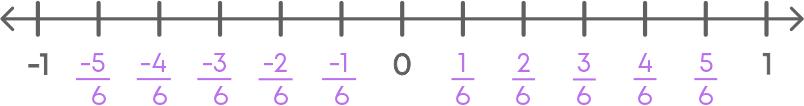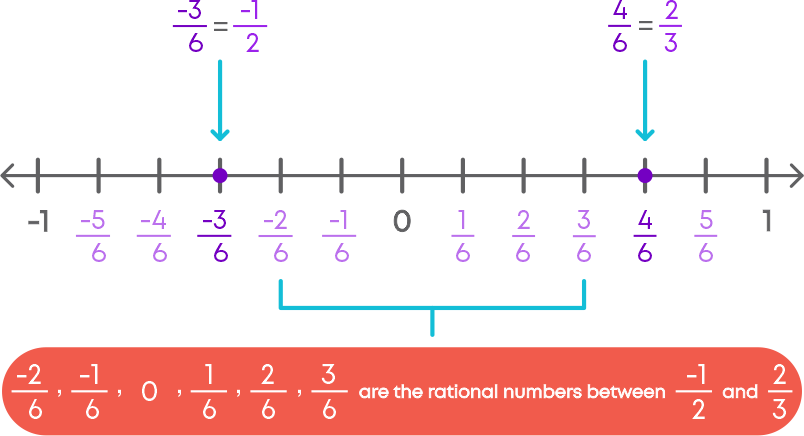Also, she knows that -1×6 2×6 = -6 12 and 2×4 3×4 = 8 12 are also equivalent rational numbers.

Now, she plots these on the number line.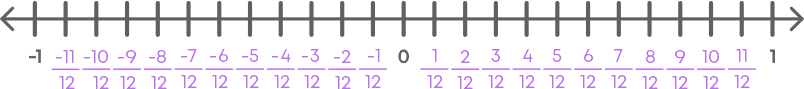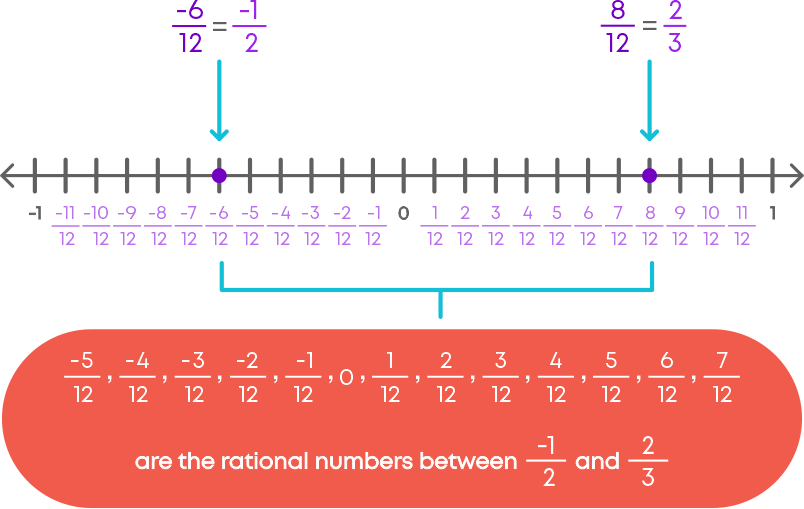By continuing this process, Sana can get an unlimited number of equivalent rational numbers of the given rational numbers. In other words, Sana realised that the list is unending. This means that she can get an unlimited number of rational numbers between two given rational numbers.

There can be infinite number of rational numbers between two rational numbers.

To find how many rational numbers are between two rational numbers, we follow these steps:

• Consider two given rational numbers.
• Convert each of the rational number to have the same denominator which is the LCM of the denominators.
• To find more rational numbers, we convert the denominators to a higher multiple of the LCM and find the equivalent rational numbers.

#### Operations On Rational Numbers

We have already learnt addition of like fractions. While adding like fractions, we just add the numerators. What about the denominator? The denominator remains the same as the given fractions.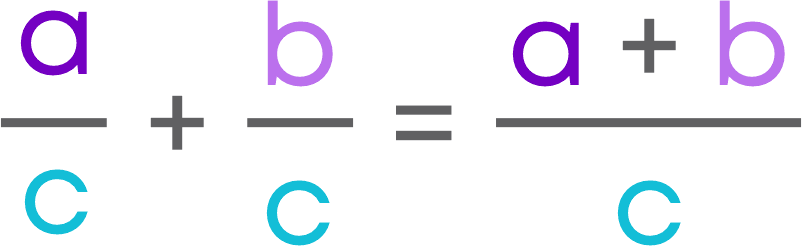In the same way, we can add two rational numbers too. Consider the two rational numbers, which have the same denominator: 13 6 and 11 6

• Add the numerators and keep the denominator same.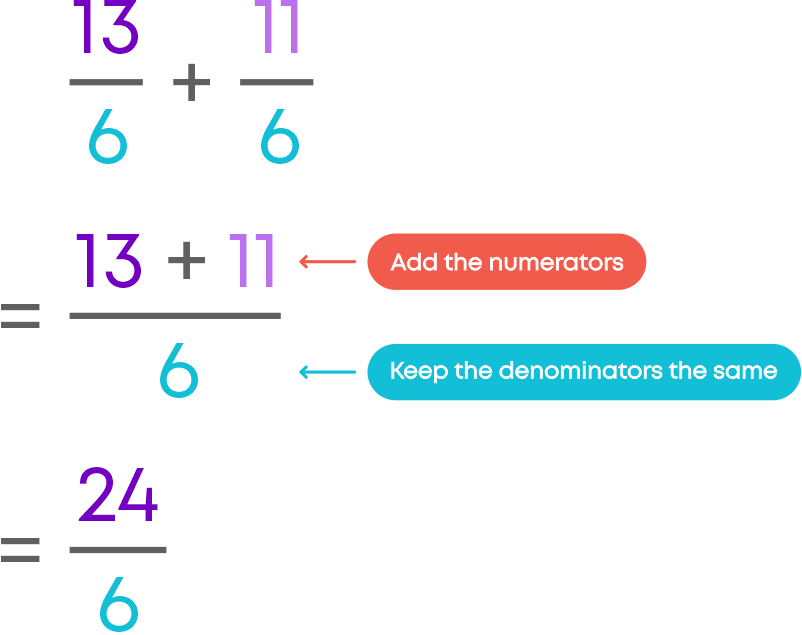Now, consider two rational numbers which have different denominators: 3 7 and 2 3

LCM of 7 and 3 is 21

3×3 7×3 = 9 21 and 2×7 3×7 = 14 21

9 21 + 14 21 = 23 21

Hence, 37 + 23 = 23 21

Note that while adding the negative rational numbers the signs should be taken care of.

We know that the additive inverse of an integer ‘a’ is the integer that, when added to ‘a,’ gives 0. This number is also called as the negative of the integer, i.e., a + (-a) = 0.

Let us learn the additive inverse of a rational number.

• The additive inverse of a rational number is its negative.
• The sum of a rational number and its additive inverse is zero.

Consider the rational number 3 4

The additive inverse of 3 4 is -3 4

3 4 + ( -3 4 ) = 0

The additive inverse of a rational number is its negative.

We know that subtraction is just the addition of additive inverse of the number to be subtracted. For example, subtracting 5 from 7 is equivalent to adding the additive inverse of 5 to 7, i.e., 7 – 5 = 7 + (-5) = 2

Raju and Rita are solving problems on rational numbers.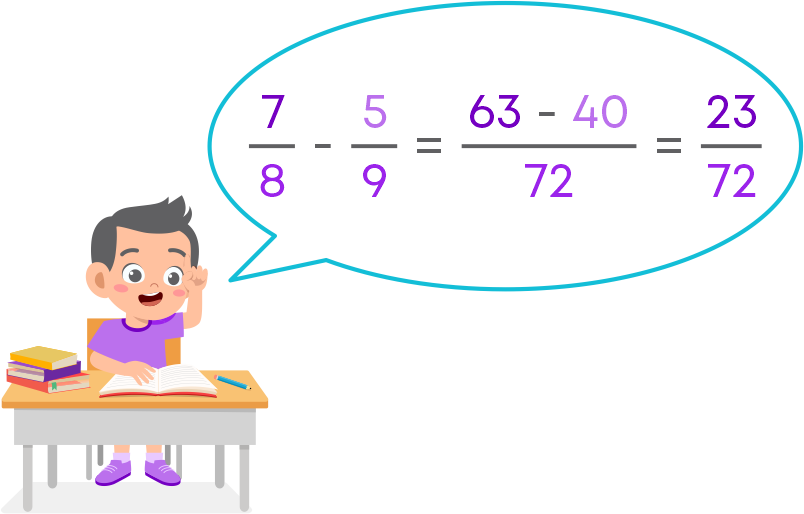Raju knows that the fractions are rational numbers, so he subtracted them just like fractions.

Now, Rita subtracted this in a different way.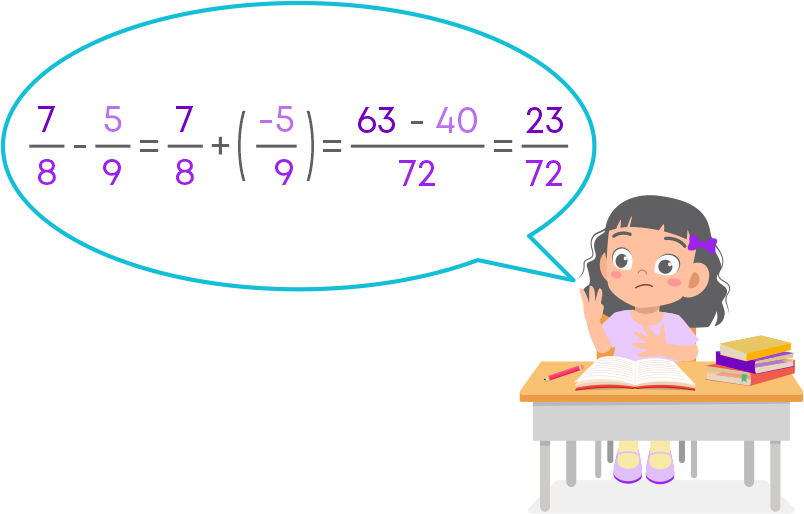Rita knows that subtraction is the same as adding the additive inverse. So, she has done the subtraction in this way.

We can see that both got the same answer. Hence, we can say that subtraction of two rational numbers is same as the addition of additive inverse of the number to be subtracted.

Also, we can subtract the rational numbers using the following steps:

1. Find the LCM of the denominators of the rational numbers.
2. Find rational numbers equivalent to given rational numbers, with LCM as the denominator.
3. Subtract the numerators of the equivalent rational numbers and keep denominator same.

We know how to multiply a fraction by a whole number. To multiply a fraction by a whole number, we multiply the given whole number and the numerator of the fraction while keeping the denominator same. In the same way, we can multiply a rational number with an integer. Now, consider a rational number 4 23 and an integer 9.

Let us multiply a rational by an integer.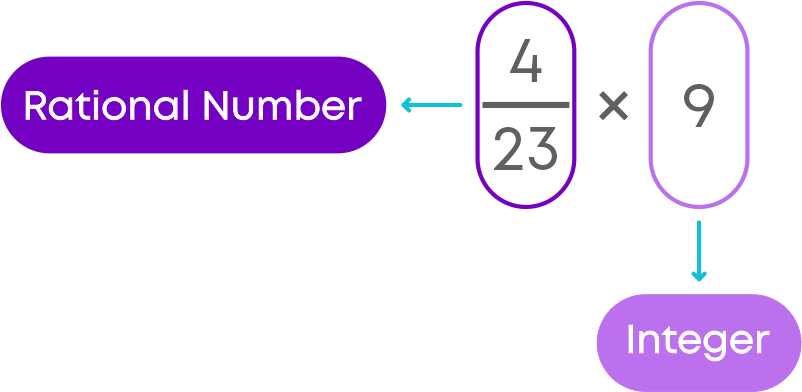Multiply the numerator of the rational number by the integer and keep the denominator the same.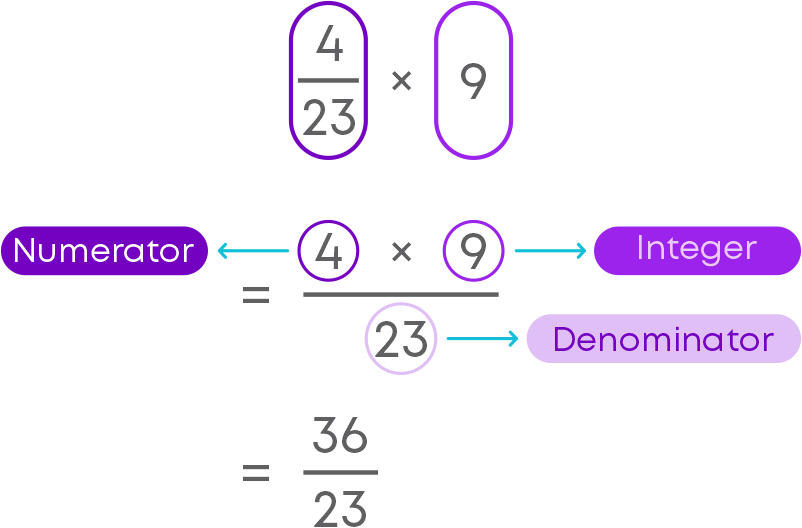We know how to multiply a fraction by another fraction. To multiply a fraction by another fraction,

• Multiply the numerator of the 1st fraction with the numerator of the 2nd fraction.
• Multiply the denominator of the 1st fraction with the denominator of the 2nd fraction.

In the same way, we can multiply a rational number by a rational number. Now, consider rational numbers -3 4 and 1 7 .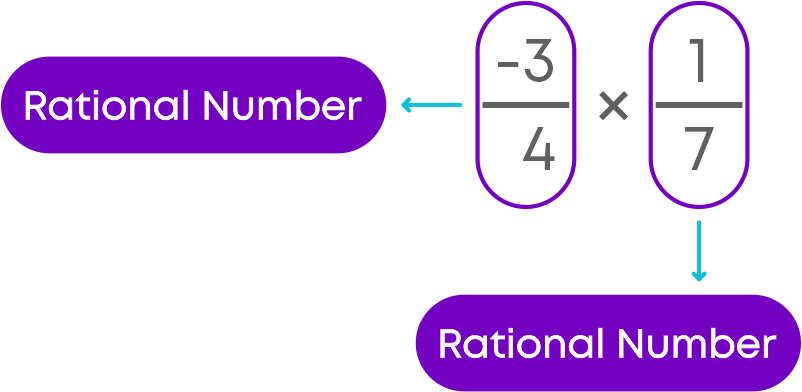• Multiply the numerator of the 1st rational number with the numerator of the 2nd rational number.
• Multiply the denominator of the 1st rational number with the denominator of the 2nd rational number.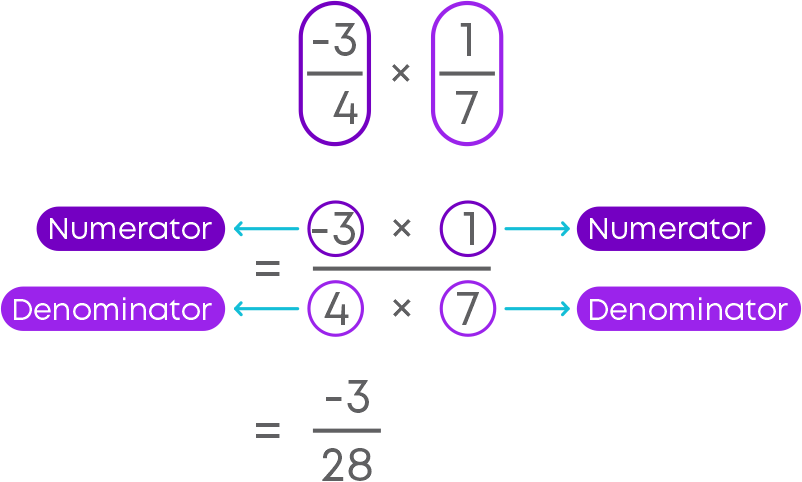You know how to write the reciprocal of fractions. In the same way, we can write reciprocal of a rational number. Let us take a rational number 13 5 .

Now, interchange the numerator and denominator of the given rational number.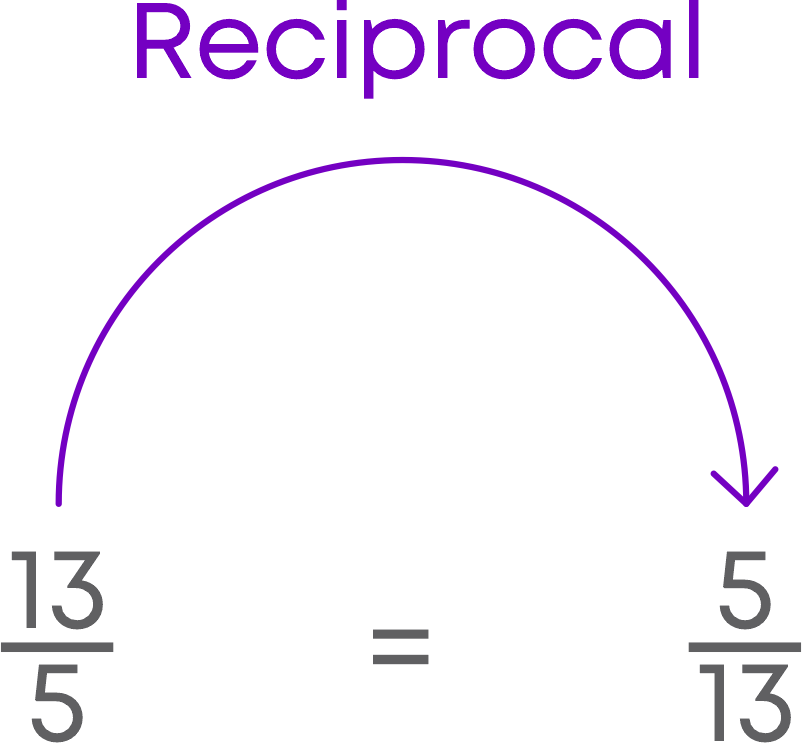So, reciprocal of 13 5 = 5 13

Hence, we can conclude that reciprocal of a rational number is obtained by interchanging the numerator and denominator of the given rational number.

You know that the reciprocal of the given rational number is obtained by interchanging the numerator and denominator. Consider the rational number and its reciprocal, -5 7 and -7 5 .

Product of rational number and its reciprocal = -5 7 × -7 5 = 1

So, we can conclude that the product of a rational number and its reciprocal is 1.

While dividing a fraction by the other, we multiply the dividend fraction by the reciprocal of the divisor fraction. The same procedure is followed for rational numbers too. That is, to divide a rational number by another rational number, we multiply the dividend rational number by the reciprocal of the divisor rational number. Consider the rational numbers -1 8 and 5 4 . Let us divide them: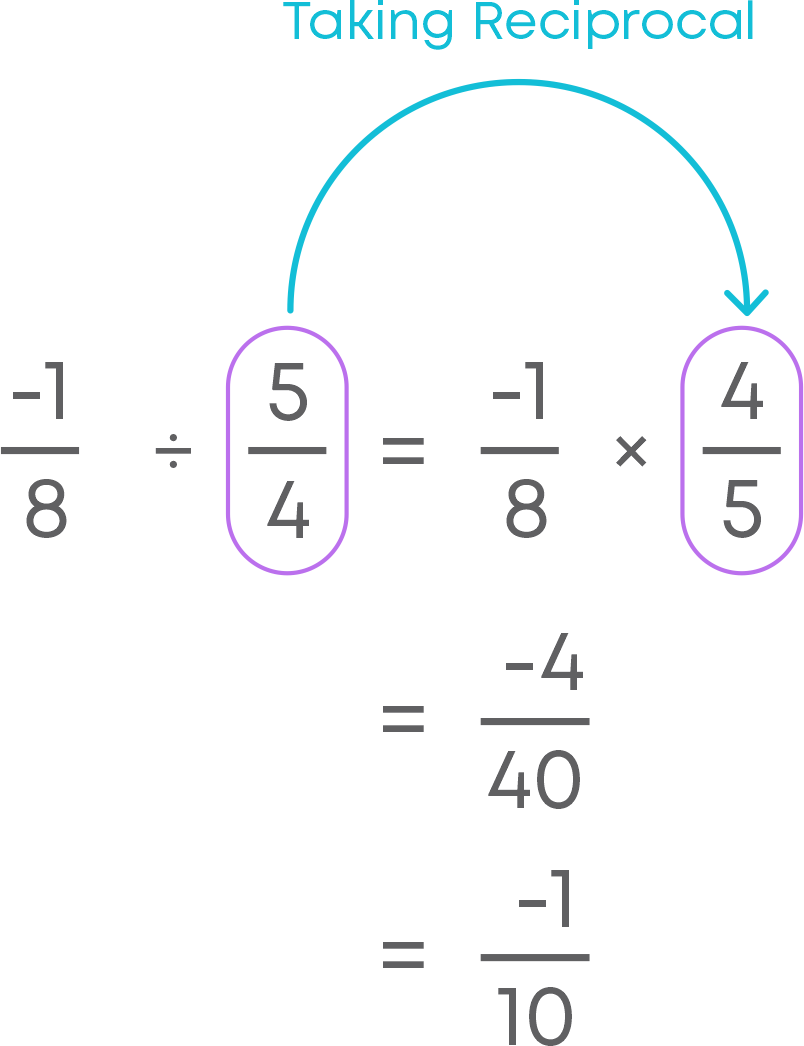Hence, -1 8 ÷ 5 4 = -1 10

#### Common Errors

The following are topics in which students make common mistakes when dealing with rational numbers:

• 1. Integers are rational numbers
• 2. Reduce rational numbers to their simplest forms
• 3. Comparing positive rational numbers
• 4. Addition of rational numbers
• 5. Subtraction of rational numbers
• 6. Cancelling out common factors
• 7. Multiplication of rational numbers with integers
• 8. Multiplication of a rational number by another rational number
• 9. Division of a rational number by another rational number

#### Integers Are Rational Numbers

A rational number is a number that can be written in the form p q , where ‘p’ and ‘q’ are integers, q≠0.

Also, every integer can be written in the form of p q , where q ≠ 0.

Hence, every integer is a rational number. For example,

Is (-22) a rational number? Consider the integer (-22). (-22) can be written as (-22) 1 . So, (-22) is a rational number.

#### Reduce Rational Numbers To Their Simplest Forms

To convert the given rational number to its simplest form we follow these steps:

• Find the HCF of the numerator and the denominator.
• Divide the numerator and the denominator by the HCF.
• The resultant rational number is the simplest form of the given rational number.

For example, let us find the simplest form of the rational number -36 96 :

HCF of 36 and 96 is 12.

(-36) 96 = -36÷12 96÷12 = -3 8

Hence, the simplest form of -36 96 = -3 8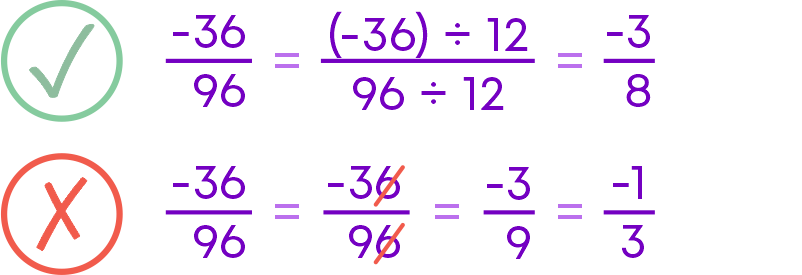#### Comparing Positive Rational Numbers

To compare positive rational numbers, we follow these steps:

• Find the LCM of the denominators of both rational numbers.
• Convert each of the rational numbers as an equivalent rational number with the denominator as the LCM.
• Compare the numerators of the equivalent rational numbers.

For example, let us compare two rational numbers 4 7 and 3 4 .

LCM of 7 and 4 is 28.

Now, 4 7 = 16 28 and 3 4 = 21 28

Since 16 < 21

16 28 < 21 28

Hence,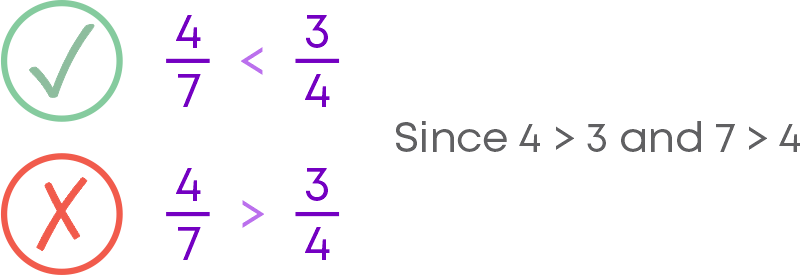• Find the LCM of the denominators.
• Convert each rational number to an equivalent rational number with the denominator as the LCM obtained.
• Add the numerators and retain the common denominator.

For example, to find the sum of -7 9 and 1 3 :

LCM of 3 and 9 is 9.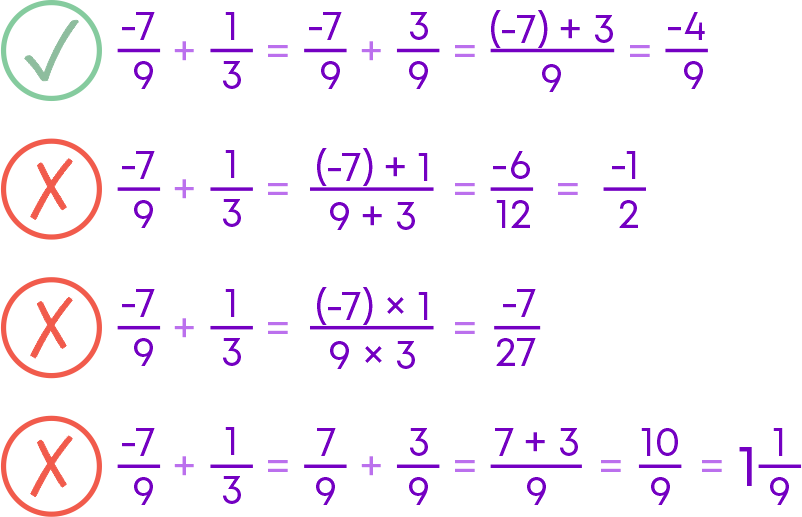While doing additions, care should be taken with the signs.

#### Subtraction Of Rational Numbers

To subtract the rational numbers,

• Find the LCM of the denominators.
• Convert each rational number to an equivalent rational number with the denominator as the LCM obtained.
• Subtract the numerators and retain the common denominator.

For example, to find 6 7 - ( -7 12 ):

LCM of 7 and 12 is 84.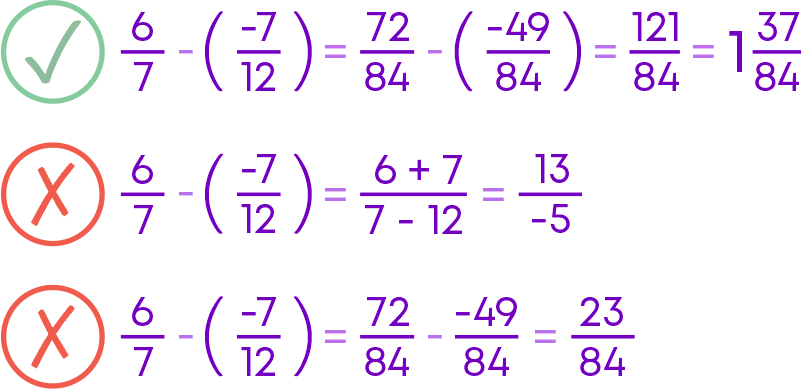While doing subtraction, care should be taken with the signs.

#### Cancelling Out Common Factors

Consider the rational number -3 8 and whole number 12. Let us multiply them -3 8 × 12 .

Here 3 and 12 have common factors but we cannot cancel them since both 12 and 3 are the numerators.

We can cancel out the common factors of 8 and 12 since 8 is in the denominator and 12 is in the numerator.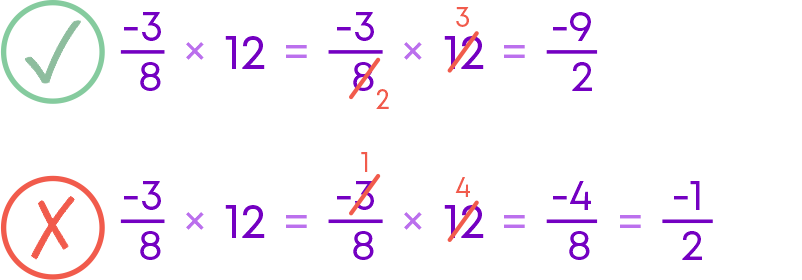#### Multiplication Of Rational Numbers With Integers

To multiply a rational number with an integer,

• Multiply the numerator of the rational number by the integer.
• Keep the denominator the same.
• Simplify the result into its simplest form.

Consider -5 12 × 4

4 can be written as 4 1

Here, we should multiply the numerator of the rational number by the integer, we cannot multiply the denominator of the rational number by the integer.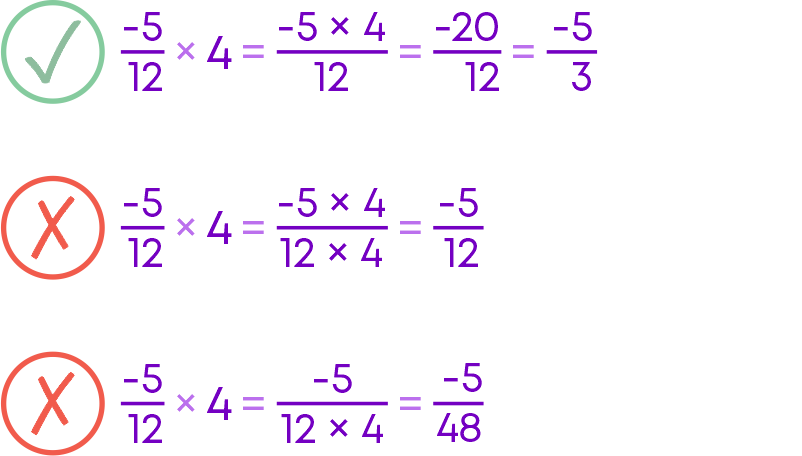#### Multiplication Of A Rational Number By Another Rational Number

To multiply a rational number by a rational number,

• Multiply the numerator of the first rational number with the numerator of the second rational number.
• Multiply the denominator of the first rational number with the denominator of the second rational number.

Consider -9 5 and -3 5

Here, we should multiply the numerator of the first rational number by the second rational number and then multiply the denominator of the first rational number by the second rational number.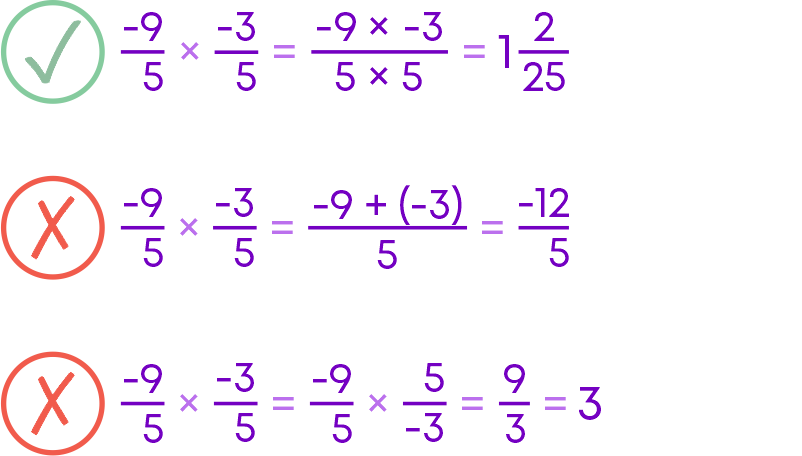Remember! Multiply the numerator with the numerator and the denominator with the denominator.

#### Division Of A Rational Number By Another Rational Number

To divide a rational number by a rational number,

• Multiply the dividend rational number by the reciprocal of the divisor rational number.

Divide -7 15 by 1 5

Division is the same as multiplying with the reciprocal of the divisor.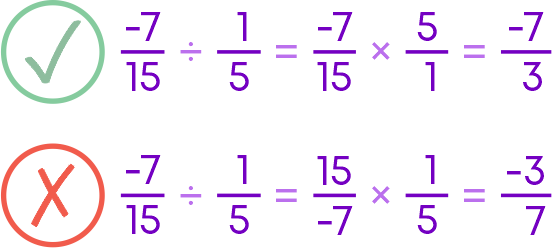#### Conclusion

Can you solve this riddle based on rational numbers?

Three kangaroos – Kang, Kong, and Roo – are in a jumping contest where 0 is the starting point.

They must jump on the right side of zero.

Use the following information to represent their progress on a number line:

(i) Roo jumped the farthest distance and won the competition.

(ii) Kang got confused and ran 52 metres in the wrong direction.

(iii) The other two jumps made were at a distance of 74 and 155 metres.

Among the options below, choose the number line which represents the final positions of the three kangaroos.

1.2.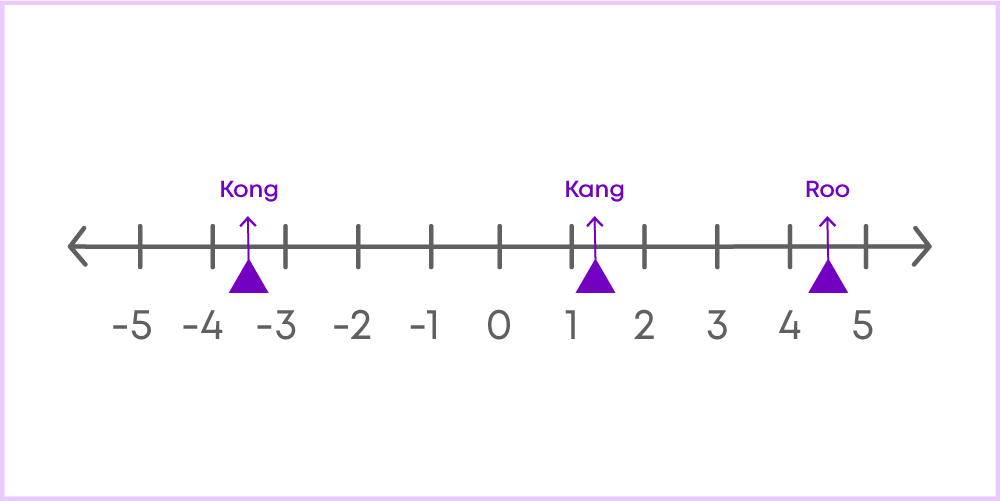3.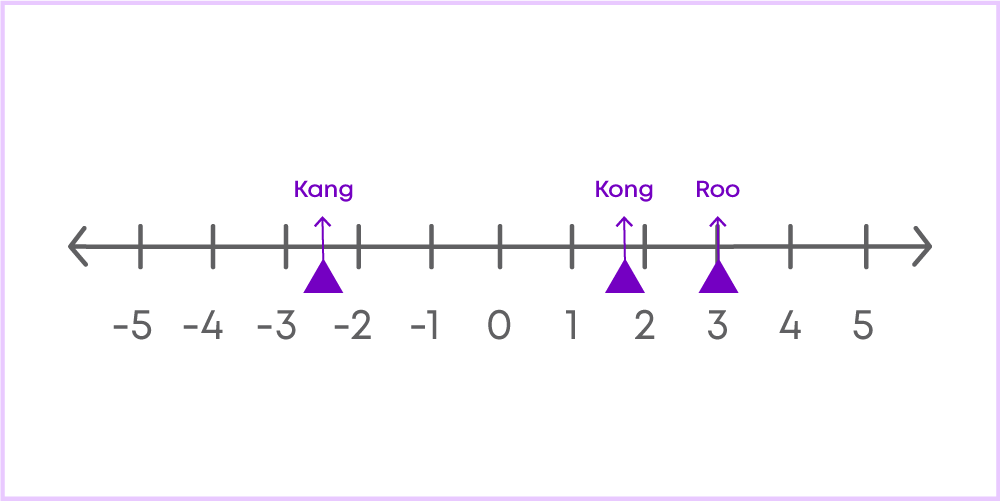4.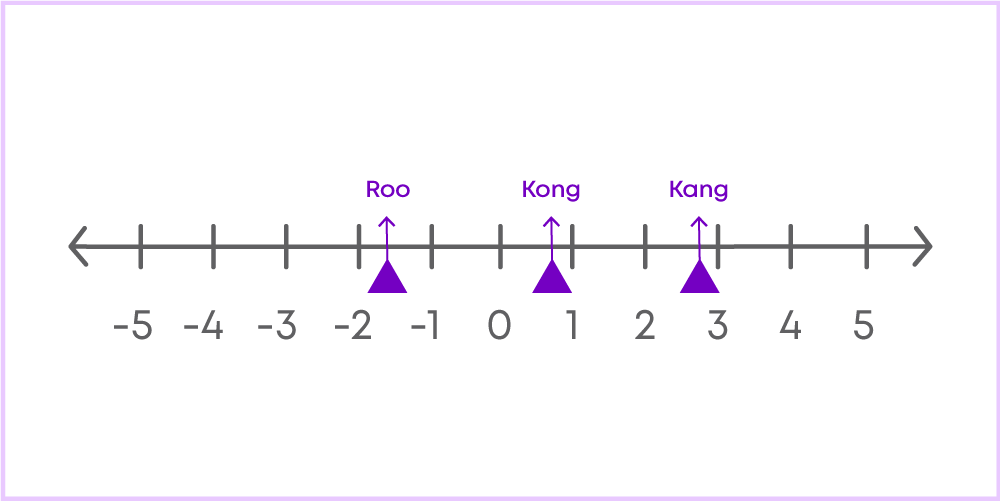#### Jyothi

Author

A math enthusiast and an educationist, Jyothi has a strong academic background and has demonstrated an impressive history of working in the education industry. Jyothi is skilled in developing and reviewing lesson plans, learning content, assessments, and video story boards and scripts.